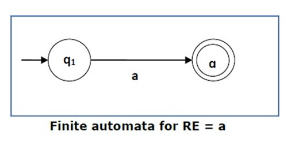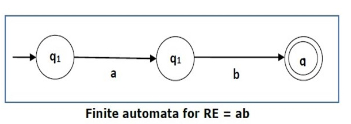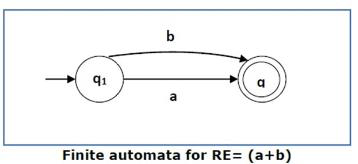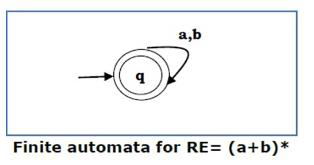# How to convert Regular expression to Finite Automata?

To convert the regular expression (RE) to Finite Automata (FA), we can use the Subset method.

Subset method is used to obtain FA from the given RE.

• Step 1 − Construct a Transition diagram for a given RE by using Non-deterministic finite automata (NFA) with ε moves.
• Step 2 − Convert NFA with ε to NFA without ε.
• Step 3 − Convert the NFA to the equivalent Deterministic Finite Automata (DFA).

Some basic RE are as follows −

Case 1 − For a regular expression ‘a’, we can construct FA as shown below −Case 2 − For a regular expression ‘ab’ we can construct FA, as given below −Case 3 − For a regular expression (a+b) we can construct FA as follows −Case 4 − For a RE (a+b)*, We can construct FA as mentioned below −